Mobile QR Code1. (Department of System Semiconductor Engineering, Sangmyung University, 31 Sangmyungdae-gil, Dongnam-gu, Cheonan, Chungnam, 31066, Korea)

Gate leakage current noise, drain current noise, nanoscale MOSFETs, ultrathin gate oxides, cross correlation effects, quantum-mechanical effects, BSIM

## I. INTRODUCTION

Aggressive downscaling of MOSFETs has been driven by significant advances in CMOS technologies, leading to increasing demands for compact model (CM) development. The compact modeling of MOS devices is critical for the computer-aided design (CAD) of digital and analog VLSI circuits (1,2). BSIM3v3 was selected as the first and public domain MOSFET model for standardization by the compact model council (CMC). Although BSIM6 has demonstrated the capability to predict sub-micron device characteristics, it is still necessary to develop a generic and practical model flexible to the significant changes in modern CMOS devices with nanometer dimensions (3). Especially, an accurate and physical noise modeling is essential for the use of modern CMOS devices, because the noise becomes increasingly larger with shrinking devices (4-7). With the downscaling of gate oxide thickness, the effects of the gate tunneling current noise become significantly critical and should thus be incorporated to accurately predict the overall noise performance (7,8). The noise models should describe all kinds of the noise sources, including the important physical effects of nanoscale MOSFET, such as quantum mechanical effects in the inversion layer (9-11).

The BSIM6 does not contain the noise model of gate leakage tunneling current as well as the correlation noise model between the gate and drain at low frequencies, which are no longer negligible in ultrathin oxide MOSFETs. In addition, BSIM6 noise model of drain current has several drawbacks which are physical limitations for trapping-detrapping process, unrealistic trap distribution over the energy and space, no capability of explaining the gate bias dependence of the frequency exponent, and no consideration of parasitic series resistance (3,12,13). In order to overcome the problems mentioned above, we have developed the physics-based compact model of the gate leakage current and noise in ultrathin gate oxide MOSFETs (7). Also, the newly proposed drain noise model can accurately predict the excess noise behavior of MOS transistors with ultrathin gate oxide (13). It was found for nitrided oxide that the results simulated by the proposed current model have better agreement with the experimental results than those under BSIM6 model. In addition, for the overall noise performance of ultrathin oxide MOSFETs at low frequencies, the correlation noise between the gate and the drain should be modeled, and the noise model has been developed (14). Furthermore, we have proposed the newly developed drain current noise model and QM correction model, based on sound physical reasoning (4).

In this paper, the physics-based compact noise model will be expressed in such a way that the models incorporate important physical effects and are suitable for circuit simulators, including QM effects on the gate leakage current noise, the drain current noise, and the correlation noise. An emphasis on the trap-related parameters of noise models is laid to make the models adaptable to the variations in different process technologies and to make its parameters easily extractable from measured data. With the help of an accurate and generally applicable compact noise model, we can successfully implement the compact noise models into BSIM format.

## II. GATE LEAKAGE CURRENT AND NOISE MODELS

### 1. Model Implementation in BSIM

The gate leakage current model is based on the inelastic trap-assisted tunneling process (ITAT). The model describes electrons tunneling to deep-lying trap states and, immediately, released to positions deeper than the original position, and subsequently tunneling to the gate (15). In terms of trap-related parameters, $X_{t}$, $\phi _{t}$, ${CCS}$, and ${NOID}$, defined in Table 1, the expressions for the gate leakage current density with the drain-to-source

Table 1. Trap-related parameters of noise model of gate leakage current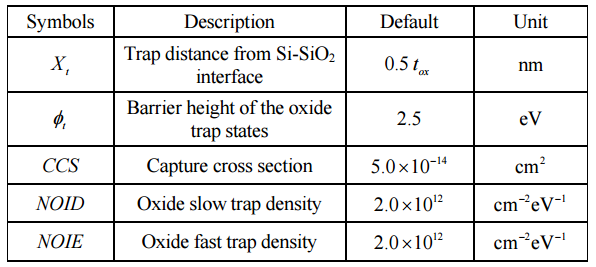voltage $V_{ds}$ = 0 $V$ are rewritten as (15)

##### (1)
$$J_{g0}=\frac{q^{2}}{16\pi ^{2}\mathrm{\hslash }\varepsilon _{ox}}\cdot CCS\cdot NOID$$ $$\times \frac{\frac{C\left(\phi _{b},X_{t},F_{ox1}\right)}{\phi _{b}}e^{- \frac{4\sqrt{2m_{ox}}}{3q\mathrm{\hslash }}\frac{\phi _{b}^{3/2}}{F_{ox1}}\beta \left(\phi _{b},X_{t},F_{ox1}\right)}}{1+\frac{\phi _{t}}{\phi _{b}}\cdot \frac{C\left(\phi _{b},X_{t},F_{ox1}\right)}{C\left(t,t_{ox}- X_{t},F_{ox2}\right)}e^{- \frac{4\sqrt{2m_{ox}}}{3q\mathrm{\hslash }}\left[\frac{\phi _{b}^{3/2}}{F_{ox1}}\beta \left(\phi _{b},X_{t},F_{ox1}\right)- \frac{\phi _{t}^{3/2}}{F_{ox2}}\beta \left(\phi _{t},t_{ox}- X_{t},F_{ox2}\right)\right]}}$$

with

##### (2a)
$\phi _{t}=\phi _{b}- qF_{ox1}X_{t}+E_{loss}$

##### (2b)
$F_{ox1}=F_{ox}+\frac{t_{ox}- X_{t}}{t_{ox}}\cdot \frac{q^{2}NOID}{\varepsilon _{ox}},\,\,\,\,F_{ox2}=F_{ox}- \frac{X_{t}}{t_{ox}}\cdot \frac{q^{2}NOID}{\varepsilon _{ox}}$

##### (2c)
$\beta \left(\phi _{b},X_{t},F_{ox1}\right)=1- \left(1- q\frac{X_{t}}{\phi _{b}}F_{ox1}\right)^{\frac{3}{2}}$

##### (2d)
$\beta \left(\phi _{t},t_{ox}- X_{t},F_{ox2}\right)=1- \left(1- q\frac{t_{ox}- X_{t}}{\phi _{b}}F_{ox2}\right)^{\frac{3}{2}}$

where ${q}$ is the electron charge, $\mathrm{\hslash }$ is the reduced Planck constant, $\varepsilon _{ox}$ is the oxide dielectric constant, $\phi _{b}$ is the Si-SiO$_{2}$ barrier height, $m_{ox}$ is the effective mass of tunneling carriers, $t_{ox}$ is the oxide thickness, $E_{loss}$ is the energy loss, $F_{ox1,2}$ is the local electric field, and ${C}$ is the correction function. The trap position$X_{t}=0.5t_{ox}$under uniform distribution extracted from a fitting procedure is consistent with the contour plot of experimental current density. The equations include the effects of charge stored in the trap states and the dependence of the energy loss on the oxide electric field (15).

The dependence of gate leakage current on the drain-source voltage is described by the source-drain partition model which has been already implemented in BSIM6 (16). The gate tunneling current is given by

##### (3)
$$I_{g}\left(V_{gs},V_{ds}\right)=\frac{J_{g0}WL\left(1- e^{- {B^{*}}KL}\right)}{B^{*}KL}$$

with

##### (4)
$$B^{*}=\frac{P_{igcd}t_{ox}}{V_{gs}^{2}}\cdot \frac{4\sqrt{2qm_{ox}}\phi _{b}^{\frac{3}{2}}}{3\mathrm{\hslash }},\,\,\,\,K=\frac{\left(V_{gs}- V_{T}- 0.5V_{ds}\right)V_{ds}}{\left(V_{gs}- V_{T}\right)L}$$

where ${W}$ is the channel width, ${L}$ is the channel length, $P_{igcd}$ is a fitting parameter with a default value of 1, $V_{T}$ is the threshold voltage, and $V_{gs}$ is the gate-to-source voltage.

One possible mechanism for $1/f^{\gamma }$ noise contribution is for traps to affect locally the barrier height or shape due to thermal noise of the frequency-dependent conductance of the oxide slow traps (17). This barrier height fluctuation (BHF) in turn modulates the tunneling transmission, and causes the fluctuation in the tunneling current. The $1/f^{\gamma }$ noise model of gate leakage current is implemented into BSIM6 with three assumptions, 1) The oxide slow traps are uniformly distributed over the energy and space, 2) the parallel trap conductance and capacitance is constant over low frequencies, and 3) the carrier tunneling is effective for trap levels around the quasi-Fermi level. Thus, the $1/f^{\gamma }$ noise model of gate leakage current can be simplified as (4,7)

with

##### (6)
$$V_{ox}=V_{gs}- V_{FB}- \psi _{s}- V_{poly}$$

where $\lambda _{t}=2\sqrt{2m_{ox}\phi _{b}}/\mathrm{\hslash }$, ${f}$ is the frequency, $C_{ox}$ is the oxide capacitance, $V_{ox}$ is the oxide voltage, $\psi _{s}$ is the surface potential, $V_{FB}$ is the flat-band voltage, and $V_{poly}$ is the polysilicon voltage drop.

The mechanism for shot noise associated with generation-recombination process can be explained by carrier transport based on trap-assisted tunneling (7,18,19). The generation rate, i.e., the transition rate from the substrate ($g_{st})$ and the gate ($g_{gt})$ to the unoccupied trap in the oxide, and recombination rate, i.e., the transition rate from the occupied trap to the substrate ($r_{ts})$ and the gate ($r_{tg})$, are caused by the statistical occupancy fluctuation of fast oxide traps in the oxide. The time constant $\tau _{fast}$ is associated with the rate equation for carrier number fluctuation $\Delta N$ with $\tau _{fast1}$ and $\tau _{fast2}$being the time-constants related to the variation of the generation-recombination rate due to the carrier number fluctuations through the substrate and gate, respectively (7). Making a Fourier analysis of the fluctuations $\Delta r_{tg}$ in the recombination rate and $\Delta g_{st}$ in the generation rate and Langevin equation for $\Delta N$, the Lorentzian-modulated(LM) shot noise model is given by (7)

##### (7)
$$S_{I_{g}}^{shot}\left(f\right)=2qI_{g}\cdot F^{Fano}$$

with

##### (8a)
$$F^{Fano}=1- \frac{2}{\tau _{fast2}}\cdot \frac{\tau _{fast}}{1+4\pi ^{2}f^{2}\tau _{fast}^{2}}+\frac{2}{\tau _{fast2}^{2}}\cdot \frac{\tau _{fast}^{2}}{1+4\pi ^{2}f^{2}\tau _{fast}^{2}}$$

##### (8b)
$\frac{1}{\tau _{fast}}=\frac{1}{\tau _{fast1}}+\frac{1}{\tau _{fast2}}$

##### (8c)
$\frac{1}{\tau _{fast1}}=\pm 0.5\cdot q\cdot CCS\cdot NOIE\cdot \frac{\partial g}{\partial N}$

##### (8d)
$\frac{1}{\tau _{fast2}}=\pm 0.5\cdot q\cdot CCS\cdot NOIE\cdot \frac{\partial r}{\partial N}$

where $F^{Fano}$ is the Fano factor, $\tau _{fast1,2}$ is the local time constants, ${N}$ is the total number of carriers, and ${g}$ and ${r}$ are the uniform generation and recombination rate, respectively. The signs of Eq. (8c) and (8d) depend on

Fig. 1. Enhancement and suppression in the Fano factor, depending on the signs of local time constants ($f$ = 10 kHz).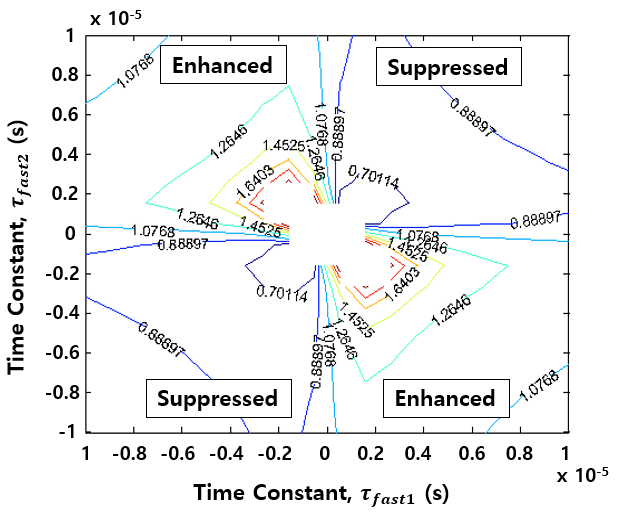the positive or negative correlation between tunneling current components. As illustrated in Fig. 1, for example, different signs of the time constants lead to enhanced shot noise. The Fano factor for nitrided oxide nMOSFETs was simulated with ${f}$ = 10 kHz, $t_{ox,eq}=2.2\,\,\mathrm{nm}\,, $$\varepsilon _{ox}=5.7\varepsilon _{0},$$\phi _{b,ox}=2.6\,\,\mathrm{eV}\,, $$m_{ox}=0.4m_{0}, and NOIE=2\times 10^{12}\,\,\mathrm{cm}^{- 2}\mathrm{eV}^{- 1}at {T} = 300 K. Due to the quantized energy levels in the inversion layer, the peak charge density is situated at a distance away from the Si-SiO_{2} interface (4). This results in the enhancement in the oxide thickness and the reduction in the oxide voltage. Therefore, the quantum effects are taken into account by using the correction for the oxide thickness, t_{ox}^{QM}, and the oxide voltage, V_{ox}^{QM}, as follows ##### (9a) t_{ox}^{QM}=t_{ox}+\frac{\varepsilon _{ox}}{\varepsilon _{si}}x_{n}^{QM}\left(\psi _{s}\right) ##### (9b) V_{ox}^{QM}=V_{gs}- V_{FB}- \psi _{s}^{QM}- V_{poly} where x_{n}^{QM} is the average centroid position from the interface and \psi _{s}^{QM} is the QM corrected surface potential. ### 2. Benchmark Tests For the practical application of the compact noise model of gate leakage current, the models are implemented into BSIM6 in public domain, as shown in Fig. 2. Implementation of the gate leakage current and noise models into the BSIM public domain platform.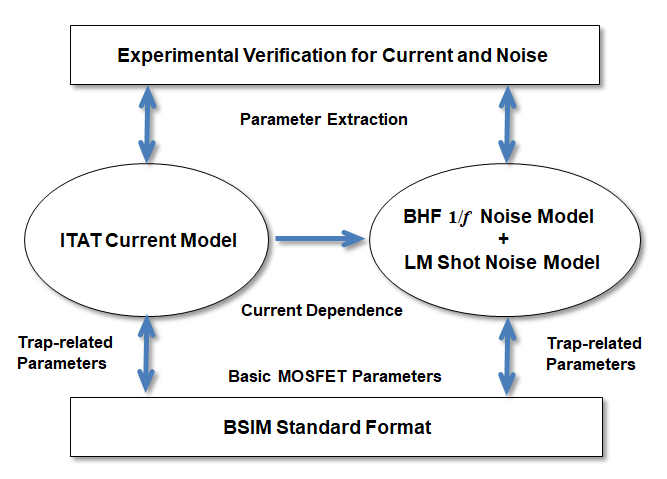Fig. 2. The basic device parameters are also used for the current and noise models, but the trap-related parameters are newly introduced into simulators. The validation for the compact model is derived from the comparisons of simulation results and measurements. nMOSFETs used for measurements were fabricated with using a manufacturable remote plasma nitride oxide (RPNO) process (15). The gate oxide was grown with both furnace and rapid-thermal based processes. Nitridation was performed by exposing the gate oxide to a short, high density, remote helicon-based nitrogen discharge. Following N_{2}-plasma nitridation and post-nitridation anneal of the gate oxide, an undoped poly-Si gate layer was deposited. For n+ gates, P was implanted, and rapid-thermal annealing was then performed in N_{2} for different durations. All tests are to be done using a test circuit of a single MOSFET and an input deck in SPICE format is provided in Fig. 3(a). The models have been implemented through b6noi.c file of BSIM6 which is C code with higher efficiency. As described in Fig. 3(b), several functions are provided to calculate the gate leakage current, 1/{f} gate current noise, gate shot noise, and 1/{f} drain current noise. Fig. 4(a) shows the comparison of the test results and measured data of gate leakage current as a function of drain-source voltage, and the current density versus gate-source voltage for the inset. The test for nitrided oxide nMOSFETs was performed by using W=10\mu \mathrm{m}\,, L=10\mu \mathrm{m}\,, t_{ox,eq}= 2.2 nm, \varepsilon _{ox}=5.7\varepsilon _{0}, \phi _{b,ox}=2.6\,\,\mathrm{eV}, m_{ox}=0.4m_{0}, and NOID=NOIE=2\times 10^{12}\,\,\mathrm{cm}^{- 2}\mathrm{eV}^{- 1}at {T} = 300 K. For different gate oxide processing conditions, the trap Fig. 3. (a) Input deck, (b) implemented b6noi.c file of BSIM to simulate the gate leakage current noise of a single MOSFET for benchmark test.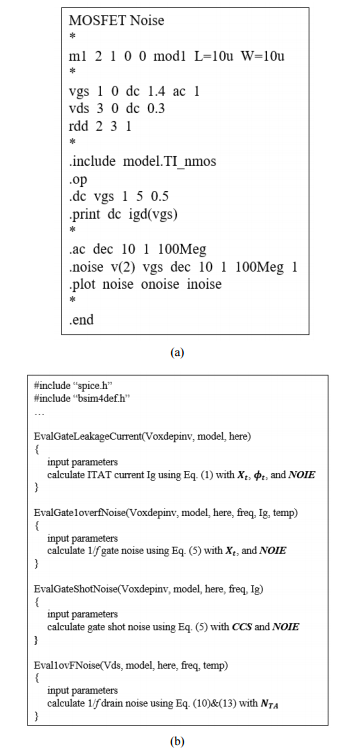density parameters (NOID,NOIE) are technology dependent. The thermal oxide devices have a trap density level one to two orders of magnitude lower than that of nitrided oxide devices (20). It is observed that the Fig. 4. Comparison of the benchmark test results and measured data (a) the gate leakage current versus V_{ds} for different V_{gs} and the current density versus V_{gs} for the inset, (b) the noise characteristics as a function of f for different V_{gs} (W=10\mu \mathrm{m}\,,$$L=10\mu \mathrm{m}\,,$$t_{ox,eq}=2.2\,\,\mathrm{nm}\,,$$NOID=NOIE=2\times 10^{12}$ $\mathrm{cm}^{- 2}\mathrm{eV}^{- 1}$).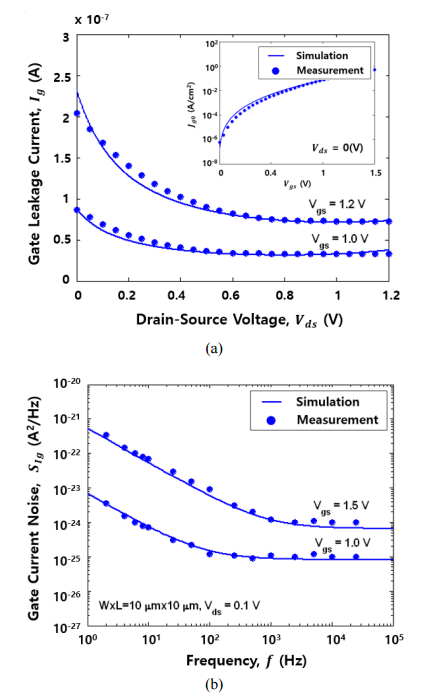simulation results have good agreement with measurements. Also, Fig. 4(b) illustrates the noise characteristics as a function of frequency for different gate-source voltages. It is also verified that the compact noise model can accurately predict the noise behavior over the frequency and bias ranges.

## III. DRAIN CURRENT AND COMPACT NOISE MODELS

### 1. Drain Current Noise Model

In order to explain the $1/f^{\gamma }$ noise of drain current in ultrathin oxide MOSFETs, the tunneling assisted-thermally activated (TATA) time constant model is employed, which is physically consistent with the gate leakage tunneling process (7,15,21). Since the noise is strongly affected by trap-related properties, the noise model accounts for a realistic description of trap distribution. In addition, the model should include the effects of the two-dimensional electric fields, the parasitic resistance, the doping profile, and the mobility degradation on the noise behavior (13). The QM correction noise model can be obtained by accounting for the QM effects through the in the oxide capacitance, the threshold voltage, and the drain current in the charge density parameters as well as the gate oxide thickness (4). The total noise power spectral density at low frequencies is obtained by calculating the correlated fluctuations in the channel carrier number and mobility and performing the integration over the channel length (4,12,13). The QM correction model for $1/f^{\gamma }~$drain current noise in the linear region, accounting for all effects mentioned above, is given by (4,13)

##### (10)
$$S_{I_{d,lin}}^{LF}\left(f\right)=\frac{qkT\cdot I_{d}^{QM}\cdot t_{ox}^{QM}}{WL^{2}\varepsilon _{ox}\left(1- \frac{V_{gs}- V_{T0}}{4\phi _{b}/q}\right)\lambda _{tun,eff}\left(0.5+k_{bulk}\right)\cdot }$$ $$\times \frac{\left[N_{TA}A^{*}\left(Q_{n1,2}^{QM},Q_{a,b}^{QM}\right)+N_{TB}B^{*}\left(Q_{n1,2}^{QM},Q_{a,b}^{QM}\right)++N_{TC}C^{*}\left(Q_{n1,2}^{QM},Q_{a,b}^{QM}\right)\right]}{\left[2\pi \tau _{eff0}\exp \left(\lambda _{th,eff}E_{fn}\right)\right]^{\left(\gamma - 1\right)}\cdot f^{\gamma }\cdot \cos \left[\frac{\pi }{2}\left(\gamma - 1\right)\right]}$$

##### (11a)
$Q_{n1}^{QM}=C_{ox}^{QM}\left(V_{gs}- R\cdot I_{d}^{QM}- V_{T0}^{QM}\right)$

##### (11b)
$$Q_{n2}^{QM}=C_{ox}^{QM}\left(V_{gs}- R\cdot I_{d}^{QM}- V_{T0}^{QM}- \left(0.5+k_{bulk}\right)\left(V_{ds}- 2R\cdot I_{d}^{QM}\right)\right)$$

##### (11c)
$Q_{a}^{QM}=- 2\left[\frac{\varepsilon _{Si}}{\beta _{1}}+C_{ox}^{QM}\left(V_{gs}- R\cdot I_{d}^{QM}- V_{FB}- \psi _{s}\right)\right],\,\,\,\,Q_{b}^{QM}=- \frac{\beta _{2}I_{d}^{QM}}{\mu _{n0}W}$

where $I_{d}^{QM}$ is the QM corrected drain current, $V_{T0}$ is the threshold voltage with $V_{ds}$= 0 V, $k_{bulk}$ is the bulk charge parameter, ${R}$ is the parasitic resistance, $\beta _{1,2}$ are the mobility parameters, $\mu _{n0}$is the maximum mobility in the inversion layer, $\tau _{eff0}$ is an effective attempt time constant, and $\lambda _{th,eff}$ and $\lambda _{tun,eff}$ are an exponential factor of thermal activation process and tunneling process, respectively. $N_{TA}$, $N_{TB}$, and$N_{TC}$are trap-related parameters, which are attributed to the noise sources for$1/f^{\gamma }$drain current noise. These parameters are different from the oxide trap density (${NOID}$) contributing to$1/f^{\gamma }$gate current noise, resulting from the partial correlation of the low frequency noise sources between the drain and the gate (7,14). Unlike BSIM6 noise model, $A^{*}$, $B^{*}$, and $C^{*}$ are not empirical constants but are analytical expressions as a function of $V_{gs}$ and $V_{ds}$(13).

In the saturation region, the noise power spectral density is expressed as the sum of the noise components due to the triode region, $L- L_{sat}$, and the pinch-off region, $L_{sat}$, and is given by integrating over each region (4,12,13)

##### (12)
$$S_{I_{d,sat}}^{LF}\left(f\right)=\left.S_{I_{d,lin}}^{LF}\left(f\right)\right| _{{V_{d}}={V_{dsat}}}+S_{I_{d,Lsat}}^{LF}\left(f\right)~$$ $$S_{I_{d},Lsat}^{LF}\left(f\right)=\frac{qkT\cdot \left(I_{d}^{QM}\right)^{2}}{WL^{2}\left(1- \frac{V_{gs}- V_{T0}}{4\varphi _{b}/q}\right)\lambda _{tun,eff}}$$

##### (13a)
$\times \frac{\left[N_{TA}A_{Lsat}^{*}\left(Q_{n,sat}^{QM}\right)+N_{TB}B_{Lsat}^{*}\left(Q_{n,sat}^{QM}\right)+N_{TC}C_{Lsat}^{*}\left(Q_{n,sat}^{QM}\right)\right]\cdot L_{sat}}{\left[2\pi \tau _{eff0}\exp (\lambda _{th,eff}E_{fn})\right]^{\left(\gamma - 1\right)}\cdot f^{\gamma }\cdot \cos \left[\frac{\pi }{2}\left(\gamma - 1\right)\right]}$

##### (13b)
$Q_{n,sat}^{QM}=C_{ox}^{QM}\left(V_{gs}- RI_{d}^{QM}- V_{T0}^{QM}- \left(0.5+k_{bulk}\right)\left(V_{dsat}^{QM}- 2RI_{d}^{QM}\right)\right)$

where $V_{dsat}$ is the drain saturation voltage, and $A_{Lsat}^{*}$, $B_{Lsat}^{*}$, and $C_{Lsat}^{*}$ are analytically expressed as a function of $V_{gs}$ and $V_{dsat}$.

### 2. Comprehensive Noise Model

Since the gate conductance in ultrathin gate oxide MOSFETs can be substantial due to the oxide traps at low frequencies, it is observed that a partial correlation between the drain and the gate current noise sources exists (7,14). In order to accurately predict the noise behavior at low frequencies, the comprehensive noise performance is evaluated by taking into account the cross correlation coefficient, $C^{LF}\left(f\right)$, between the drain and the gate current noise, and is expressed as a function of the current ratio, $\lambda _{p}=I_{g}/I_{d}$, and the noise ratio, $S_{I_{g}}^{LF}\left(f\right)/S_{I_{d}}^{LF}\left(f\right)$, as follows (14)

##### (14)
$$C^{LF}\left(f\right)=\frac{\frac{\lambda _{p}}{\left(1+\lambda _{p}\right)\left(1- \lambda _{p}\right)}\left[1- \frac{S_{I_{g}}^{LF}\left(f\right)}{S_{I_{d}}^{LF}\left(f\right)}\right]+\frac{1}{1+\lambda _{p}}\left[\frac{S_{I_{g}}^{LF}\left(f\right)}{S_{I_{d}}^{LF}\left(f\right)}\right]}{\sqrt{\left\{\begin{array}{l} \frac{\lambda _{p}^{2}}{\left(1+\lambda _{p}\right)^{2}\left(1- \lambda _{p}\right)^{2}}\left[1- \frac{S_{I_{g}}^{LF}\left(f\right)}{S_{I_{d}}^{LF}\left(f\right)}\right]^{2}+\frac{1}{\left(1+\lambda _{p}\right)^{2}}\left[\frac{S_{I_{g}}^{LF}\left(f\right)}{S_{I_{d}}^{LF}\left(f\right)}\right]^{2}\\ +\frac{\left(1+\lambda _{p}^{2}\right)}{\left(1+\lambda _{p}\right)^{2}\left(1- \lambda _{p}\right)}\left[\frac{S_{I_{g}}^{LF}\left(f\right)}{S_{I_{d}}^{LF}\left(f\right)}\right]\left[1- \frac{S_{I_{g}}^{LF}\left(f\right)}{S_{I_{d}}^{LF}\left(f\right)}\right] \end{array}\right\}}}$$

In addition to the influence of the correlation on the low frequency noise performance, the shot noise due to the gate leakage current, $S_{I_{g}}^{shot}\left(f\right)=2qI_{g}F^{Fano}$, has an impact on the noise behavior, and thus should be incorporated into the minimum noise figure $F_{min}^{LF}$ as follows (14,22,23)

##### (15)
$$F_{min}^{LF}\left(f\right)=1+\frac{S_{I_{d}}^{LF}\left(f\right)}{2kTg_{mg}^{2}}\sqrt{\left(2\pi fC_{gs}\right)^{2}\left[1- \left(C^{LF}\right)^{2}\right]\cdot \left[\frac{g_{mg}}{g_{g}}\frac{S_{I_{g}}^{LF}\left(f\right)}{S_{I_{d}}^{LF}\left(f\right)}\right]+\frac{2qF^{Fano}I_{g}g_{mg}^{2}}{S_{I_{d}}^{LF}\left(f\right)}}$$

where $g_{mg}=\partial I_{d}/\partial V_{gs}$ is the transconductance, $g_{g}=$ $\partial I_{g}/\partial V_{gs}$ is the gate conductance, and $C_{gs}$ is the gate-to-source capacitance.

### 3. Simulation Results

From the viewpoint of compact noise modeling, the model should provide an accurate prediction of noise behavior, describing all the important physical effects without an increase of parameters. For nMOSFETs with ultrathin oxide (=2.2 nm), the $1/f^{\gamma }$ noise of drain current can be accurately predicted by the expressions of Eq. (10)-(13). Fig. 5 shows the comparison of measured data and simulation results carried out with single trap-related parameter under the assumption of uniform trap distribution in the oxide. The simulation was carried out with $t_{ox,eq}=2.2\,\,\mathrm{nm}\,,$ $V_{T0}=0.45\,\,\mathrm{V},$ $k_{bulk}=0.625,$ $R=10\,\,\Omega \,,$ $\mu _{n0}=550\,\,\mathrm{cm}^{2}\mathrm{V}^{- 1}\mathrm{s}^{- 1}$and$N_{TA}=1\times 10^{18}$ $\mathrm{cm}^{- 3}\mathrm{eV}^{- 1}$at ${T}$ = 300 K. Excellent agreements between measurement and simulation are observed over the frequency and in both the linear and saturation regions for two channel lengths, as shown in Fig. 5(a) and (b), respectively.

Fig. 5. Comparison of the simulation results and measured data of the drain current noise (a) the noise characteristics as a function of frequency for two channel lengths, (b) the noise characteristics as a function of $V_{ds}$ for two channel lengths ($t_{ox,eq}=2.2$nm, $N_{TA}=1\times 10^{18}\mathrm{cm}^{- 3}\mathrm{eV}^{- 1}$).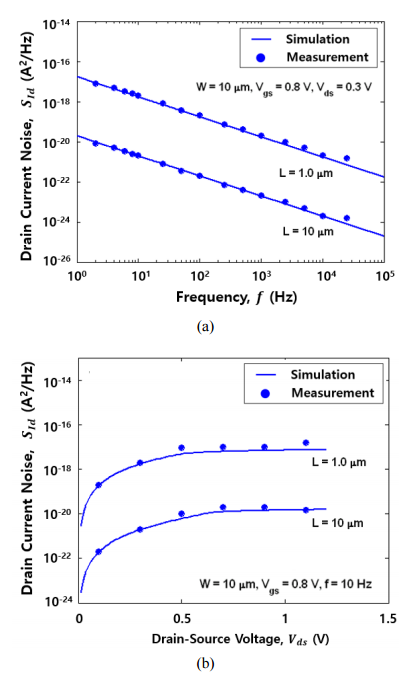For the overall noise evaluation of advanced CMOS devices, the compact noise models at low frequencies should include the correlation effects between the gate and the drain, together with the gate and drain current noise models. Unlike the capacitive coupling at high frequencies, the low frequency correlation is mainly attributed to the trap-assisted tunneling process, which is

Fig. 6. Simulation of the minimum noise figure (a) the characteristics of minimum noise figure as a function of frequency for different $V_{gs}$, (b) the characteristics of minimum noise figure as a function of $V_{gs}$ for different $V_{ds}$ ($t_{ox,eq}=2.2$nm, $N_{TA}=1\times 10^{18}\mathrm{cm}^{- 3}\mathrm{eV}^{- 1}$, $NOID=2\times 10^{12}\mathrm{cm}^{- 2}\mathrm{eV}^{- 1}$).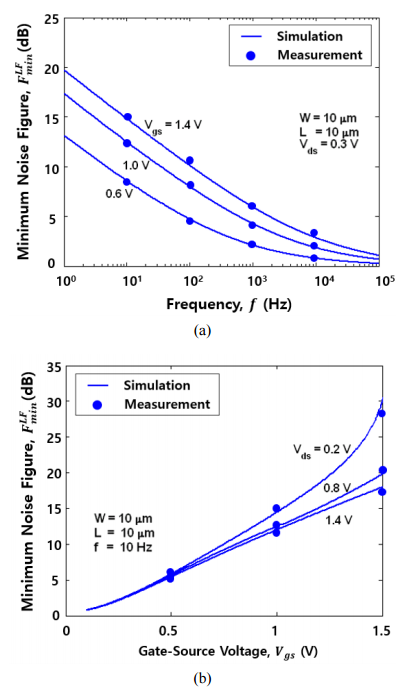a common origin of the gate and drain current noise. With the help of an analytical expression of correlation coefficient, it is possible to evaluate the minimum noise figure, as illustrated in Fig. 6. The simulation was performed by using $W=10\mu \mathrm{m}\,,$ $L=10\mu \mathrm{m}\,,$ $t_{ox,eq}=$ $2.2\,\,\mathrm{nm}\,,$ $N_{TA}=1\times 10^{18}\,\,\mathrm{cm}^{- 3}\mathrm{eV}^{- 1}$and$NOID=2\times 10^{12}$ $\mathrm{cm}^{- 2}\mathrm{eV}^{- 1}$at ${T}$ = 300 K. Fig. 6(a) and (b) show the characteristics of minimum noise figure as a function of frequency for different gate-source voltages $V_{gs}$ and as a function of the gate-source voltage for different drain-source voltages $V_{ds}$, respectively. The increasing minimum noise figure with increasing $V_{gs}$ and decreasing $V_{ds}$ can be interpreted as being due to the gate leakage current and its noise components, greatly affecting the overall noise performance at low frequencies.

## IV. CONCLUSIONS

The comprehensive and physics-based compact noise models for advanced CMOS devices were presented. The proposed noise models offer the following unique features: 1) The models incorporate important physical effects in nanoscale MOSFETs, such as the gate leakage current and noise, the low frequency correlation effect between the drain and the gate, and the trap-related phenomena. 2) The drain current noise model was improved by including the tunneling assisted-thermally activated process, the parasitic resistance, and mobility degradation. 3) The quantum-mechanical effects on the noise characteristics have been taken into consideration, extending the applicability of the model to ultrathin oxide MOSFETs. 4) With the help of proposed noise models applicable to nanoscale MOSFETs, the noise models were implemented into general-purpose circuit simulator, providing a comprehensive evaluation of noise performance at low frequencies in advanced MOSFETs devices.

### ACKNOWLEDGMENTS

This research was funded by a 2019 research Grant from Sangmyung University.

### REFERENCES

1
Singh Y. S., 2014, BSIM6: analog and RF compact model for bulk MOSFET, IEEE Trans. Electron Devices, Vol. 61, No. 2, pp. 234-2442
Gupta C., Agarwal H., Goel R., Hu C., Chauhan Y. S., 2019, Improved modeling of bulk charge effect for BSIM-BULK model, IEEE Trans. Electron Devices, Vol. 66, No. 6, pp. 2850-28533
Dasgupta A., 2020, BSIM compact model of quantum confinement in advanced nanosheet FETs, IEEE Trans. Electron Devices, Vol. 67, No. 2, pp. 730-7374
Lee J. H., Hong D. K., 2019, Charge-based quantum correction noise model in nanoscale MOSFET, Journal of Semiconductor Technology and Science, Vol. 19, No. 1, pp. 50-625
da Silva M. B., Both T. H., Tuinhout H. P., Duijnhoven A. Z., Wirth G. I., Sholten A. J., 2019, A Compact statistical model for the low-frequency noise in halo-implanted MOSFETs: large RTN induced by halo implants, IEEE Trans. Electron Devices, Vol. 66, No. 8, pp. 3521-35266
Zhang X., White M. H., 2012, A quantum mechanical treatment of low frequency noise in high-K NMOS transistors with ultra-thin gate dielectrics, Solid-State Electron., Vol. 78, No. 12, pp. 131-1357
Lee J. H., Bosman G., Green K. R., Ladwig D., 2003, Noise model of gate leakage current in ultrathin oxide MOSFETs, IEEE Trans. Electron Devices, Vol. 50, No. 12, pp. 2499-25068
Momose H. S., et al , 1998, A study of flicker noise in n- and p-MOSFETs with ultra-thin gate oxide in the direct-tunneling regime, IEDM Tech. Dig., pp. 923-9269
Ando T., Fowler A. B., Stern F., 1982, Electronic properties of two-dimensional systems, Rev. Mod.Phys., Vol. 54, No. 2, pp. 437-67210
Huang J. Z., Chew W. C., Tang M., Jiang L., 2012, Efficient simulation and analysis of quantum ballistic transport in nanodevices with AWE, IEEE Trans. Electron Devices, Vol. 59, No. 2, pp. 932-93811
Karim M. A., Haque A., 2010, A physically based accurate model for quantum mechanical correction to the surface potential of nanoscale MOSFETs, IEEE Trans. Electron Devices, Vol. 57, No. 2, pp. 496-50212
Hung K. K., Ko P. K., Hu C., Cheng Y. C., 1990, A unified model for the flicker noise in metal-oxide-semiconductor field-effect transistors, IEEE Trans. Electron Devices, Vol. 37, No. 3, pp. 654-66513
Lee J. H., Bosman G., 2004, 1/$f^{\gamma }$ drain current noise model in ultrathin oxide MOSFETs, Fluctuation and Noise Letters, Vol. 4, No. 2, pp. l297-L30714
Lee J. H., Bosman G., 2004, Comprehensive noise performance of ultrathin oxide MOSFETs at low frequencies, Solid-State Electron., Vol. 48, No. 1, pp. 61-7115
Lee J. H., Bosman G., Green K. R., Ladwig D., 2002, Model and analysis of gate leakage current in ultrathin nitride oxide MOSFETs, IEEE Trans. Electron Devices, Vol. 49, No. 7, pp. 1232-124116
Cao K. M., Lee W. C., Liu W., Jin X., Su P., Fung S. K. H., An J. X., Yu B., Hu C., 2000, BSIM4 gate leakage model including source-drain partition, IEDM Tech. Dig., pp. 815-81817
Ghibaudo G., Roux O., Duc C. N., Balestra F., Brini J., 1991, Improved analysis of low frequency noise in field-effect MOS transistors, Phys. Stat. Sol. (a), Vol. 124, pp. 571-58118
Iannaccone G., Betti A., Fiori G., 2015, Suppressed and enhanced shot noise in one dimensional field-effect transistors, J. of Comput. Electron., Vol. 14, No. 1, pp. 94-10619
Iannaccone G., Crupi F., Neri B., Lombardo S., 2000, Suppressed shot noise in trap-assisted tunneling of metal-oxide-semiconductor capacitors, Appl. Phys.Lett., Vol. 77, No. 18, pp. 2876-287820
Jayaraman R., Sodini C. G., 1990, 1/$f$ noise interpretation of the effect of the gate oxide nitridation and reoxidation on dielelectric traps, IEEE Trans. Electron Devices, Vol. 37, No. 1, pp. 305-30921
Tewksbury T. L., Lee H. S., 1994, Characterization, modeling, and minimization of transient threshold voltage shifts in MOSFET’s, IEEE J. Solid-State Circuits, Vol. 29, No. 3, pp. 239-25222
Cappy A., 1988, Noise modeling and measurement techniques, IEEE Trans. Microwave Theory Tech., Vol. 36, No. 1, pp. 1-1023
Das M. B., 1972, FET noise source and their effects on amplifier performance at low frequencies, IEEE Trans. Electron Devices, Vol. 19, No. 3, pp. 338-348## Author

Jonghwan Lee was born in Seoul, Korea, on February2, 1969.

He received the M.S. degrees from the Inha University, Incheon, Korea, and the Ph.D. degree in electrical & computer engineering from the University of Florida, Gainesville, in 1993 and 2003, respectively.

In 2017, he joined the Department of System Semiconductor Engineering, Sangmyung University, Korea, where he is currently a Professor.

From 2003 to 2016, he was with Samsung Display Inc., Korea, as a Principal Research Engineer, where he worked on semiconductor and display devices design and development.

His current research interests include semiconductor device modeling and simulation, physics-based artificial neural network, noise modeling in nanoscale MOSFETs, display device design and process-architecture, and thermoelectric transport modeling.

He has published more than 30 refereed journal and conference papers on these topics, and holds more than 20 patents in the field of display and semiconconductor devices.